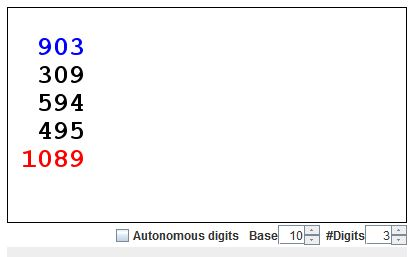# 1089 and a Property of 3-digit Numbers

Number 1089 is a centerpiece of a curious mathematical trick used to stun the uninitiated with the performer's math prowess. I was reminded about it watching David Acheson's video. David is the author of highly recommended book 1089 and all that.

Take any 3-digit number, say, 732 and write it backwards: 237. Subtract the smaller of the two numbers (237 in our case) from the larger (732). With our selection, we obtain the number 495. Write this one backwards too and compute the sum: 495 + 594. Here comes the surprise: regardless of your original selection, the final result will always be 1089!

I am going to explain shortly why this is so, but first either give it a thought or experiment with the applet below. The blue numbers are clickable. The digits increase if clicked to the right of their vertical axis, and decrease if clicked to the left of the axis.

### If you are reading this, your browser is not set to run Java applets. Try IE11 or Safari and declare the site https:///www.cut-the-knot.org as trusted in the Java setup.What if applet does not run?

Let's formulate what we've been doing - an algorithm of sorts:

For any integer A, let A' be obtained by writing A backwards. Compute B = |A - A'| and C = B + B'.

The algorithm as described conveys a general idea but, if you played with the applet, you may have noticed that the description begs for additional details. The point is that the applet (and the problem I wish to consider) deal with integers of a fixed length. Subtraction A - A' can occasionally produce numbers shorter than specified. When this happens, then B before being written backwards is padded with zero in front to insure the required number of digits. For example, B = 87, B' = 780 because the padded B is a string 087.

So the number of digits plays an important role in the procedure. To see what is going on I shall consider the integers with the specified number of digits, starting with the 1-digit numbers.

For a 1-digit A, A' = A so that B = 0 as is B' and their sum.

Now, for a 2-digit numbers A = 10a + b (1 ≤ a ≤ 9, 0 ≤ b ≤ 9), A' = 10b + a, and, assuming without loss of generality that a ≥ b, B = A - A' = 10(a - b) + (b - a). The case a = b is trivial as then B = 0. Otherwise, i.e., with a > b,

B = 10(a - b) + (b - a) = 10(a - b - 1) + (10 + b - a).

This modification is necessary because b - a is negative. The new form of B has the advantage that

0 ≤ a - b - 1 ≤ 9 and
0 ≤ 10 + b - a ≤ 9.

It follows that 10(a - b - 1) + (10 + b - a) is the decimal representation of B, so that B' = 10(10 + b - a) + (a - b - 1). Next we add:

 B + B' = 10(a - b - 1) + (10 + b - a) + 10(10 + b - a) + (a - b - 1) = 10·9 + 9 = 99,

because 10 + b - a + a - b - 1 = 9.

We see that, unless number A is palindromic, that is, read the same way either way, which happens when a = b, the algorithm always results in number 99. This is of course less exciting than getting 1089 for the 3-digit numbers but help lift the veil of the mystery shrouding the latter result.

For a 3-digit decimal number A = 100a + 10b + c, A' = 100c + 10b + a. For a palindromic number (a = c), again B = 0. To exclude this case, assume a > c, making A > A'.

B = (100a + 10b + c) - (100c + 10b + a) = 100(a - c) + (c - a).

As with the case of 2-digit numbers, a - c is a decimal digit, while c - a is negative. We apply a similar trick:

B = 100(a - c) + (c - a) = 100(a - c - 1) + 90 + (10 + c - a),

which shows that B always has 9 as the second digit while its other digits add up to 9:

(a - c - 1) + (10 + c - a) = 9.

Writing B as x9y, with x + y = 9, B' = y9x, and

 B + B' = 100(x + y) + 90 + 90 + (x + y) = 900 + 180 + 9 = 1089,

proving the magic property of the number 1089.

Every step of the derivation could be repeated in positional systems with bases other than 10. If v and u denote the largest and the penultimate digits in some base, then, for 3-digit numbers, with the exception of palindromes, the algorithm leads to 10uv. For example, in base 36 the algorithm always ends with 10YZ.

### References

1. D. Acheson, 1089 and All That: A Journey into Mathematics, Oxford University Press, 20101. 1089 and a Property of 3-digit Numbers
2. Four Digits Magic Prediction
3. Five Digits Magic Prediction